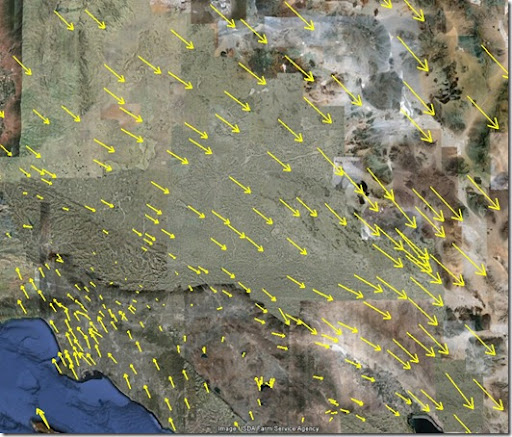## Oct 31, 2011

### How to make arrow objects for Google Earth

In the previous post, we has use GeoDestination function to make circle objects, and we can use the same function to generate arrow objects, too.

The data we have is the position and displacements in north and east direction, {lat, lon, ndis, edis}. ndis, edis is in millimeter in this case.

First, let calculator the length of the arrow, the scale here is 1 mm displacement = 1000 meters

length = Sqrt[ndis^2 + edis^2]*1000

Then the angle. GeoDestionation requires GeoDirection, it starts from the north.

angle = ArcTan[edis, ndis] /Degree
geoangle = 90 - angle

For the end point:

end = First@GeoDestination[{lat, lon}, {length, geoangle}]

The trick to generate the arrow is that to form another smaller circle around the end point, pick up two points from the arrow heads.

arr1 = First@GeoDestination[end, {dist*.25, geoangle + 180 - 30}]
arr2 = First@GeoDestination[end, {dist*.25, geoangle - 180 + 30}]

Then we can have two lines which draw in this order, {org –> end}, {arr1->end->arr2}. When comes to export kml, we need to use <MultiGeometry> object in KML, it seems not supported by Export function in Mathematica yet, so you can just export the following string directly:

<MultiGeometry>
<LineString>
<tessellate>1</tessellate>
<coordinates>
-117.093195026,34.116408002,0 -117.020521006,34.0741018797,0
</coordinates>
</LineString>
<LineString>
<tessellate>1</tessellate>
<coordinates>
-117.042636322,34.0757361767,0 -117.020521006,34.0741018797,0 -117.029869517, 34.0907835742, 0
</coordinates>
</LineString>
</MultiGeometry>

Here is the screenshot of the final product in Google Earth: